López-Beceiro, J., Álvarez-García, A., Sebio-Puñal, T., Zaragoza-Fernández, S., Álvarez-García, B., Díaz-Díaz, A., Janeiro, J., and Artiaga, R. (2016). "Kinetics of thermal degradation of cellulose: Analysis based on isothermal and linear heating data," BioRes. 11(3), 5870-5888.

#### Abstract

In spite of the many studies performed, there is not yet a kinetic model to predict the thermal degradation of cellulose in isothermal and non-isothermal conditions for the full extent of conversion. A model proposed by the authors was tested on non-oxidising thermogravimetric data. The method consisted of initially fitting several isothermal and non-isothermal curves, then obtaining a critical temperature and an energy barrier from the set of fittings that resulted from different experimental conditions. While the critical temperature, approximately 226 °C, represented the minimum temperature for the degradation process, the degradation rate at a given temperature was related to both the critical temperature and the energy barrier. These results were compared with those observed in other materials. The quality of fittings obtained was superior to any other reported to date, and the results obtained from each single curve were in line with each other.

Kinetics of Thermal Degradation of Cellulose: Analysis Based on Isothermal and Linear Heating Data

Jorge López-Beceiro, Ana Álvarez-García, Teresa Sebio-Puñal, Sonia Zaragoza-Fernández, Begoña Álvarez-García, Ana Díaz-Díaz, Julia Janeiro, and Ramón Artiaga *

In spite of the many studies performed, there is not yet a kinetic model to predict the thermal degradation of cellulose in isothermal and non-isothermal conditions for the full extent of conversion. A model proposed by the authors was tested on non-oxidising thermogravimetric data. The method consisted of initially fitting several isothermal and non-isothermal curves, then obtaining a critical temperature and an energy barrier from the set of fittings that resulted from different experimental conditions. While the critical temperature, approximately 226 °C, represented the minimum temperature for the degradation process, the degradation rate at a given temperature was related to both the critical temperature and the energy barrier. These results were compared with those observed in other materials. The quality of fittings obtained was superior to any other reported to date, and the results obtained from each single curve were in line with each other.

Keywords: Cellulose; Pyrolysis; Kinetic; Thermogravimetry

Contact information: Escola Politécnica Superior, Avda. Mendizábal s/n. 15403-Ferrol, Spain;

* Corresponding author: ramon.artiaga@udc.es

NOMENCLATURE

yramp(t) Transformation rate, as a function of time, in linear heating conditions

Cramp The peak area. Represents the amount of sample involved in each transformation process, in linear heating conditions

bramp Fitting parameter, related to the peak shape in linear heating conditions. If t=1, then brampis 4 times the maximum transformation rate per unit of sample mass

mramp The time elapsed from the beginning of the experiment to the instant where the maximum mass loss rate is observed, in linear heating experiments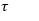Fitting parameter related to the peak asymmetry (t=1 for a symmetric peak)

yiso(t)    Transformation rate, as a function of time, in isothermal conditions

Ciso       The peak area. Represents the amount of sample involved in each transformation process, in isothermal conditions

biso       Fitting parameter, related to the peak shape in isothermal conditions. If t=1, then biso is 4 times the maximum transformation rate per unit of sample mass

miso     The time elapsed from the beginning of the experiment to the instant where the maximum mass loss rate is observed, in isothermal experiments

Tc          The critical temperature

Eiso       The true energy barrier obtained in isothermal conditions

Tp         The peak temperature, the temperature at mramp, in linear heating conditions

tpramp  The time elapsed from the instant where the T =Tc to the instant where the peak maximum is observed (T=Tp) in linear heating experiments

Eramp   An apparent energy barrier obtained in linear heating conditions

tpiso       The time from the instant at which the isotherm begins to the instant where the peak maximum is observed, in isothermal experiments

tTb         A constant representing the values of tpiso at T=Tb

Tb          A reference temperature, higher than Tc, at which the reaction would be relatively fast.

bTb        A constant representing the values of bpiso at T=Tb

INTRODUCTION

Cellulose is one of the three main components of biomass and represents approximately 30% to 50% of the weight in lignocellulosic biomass, depending on the type of biomass (McKendry 2002). Biomass and cellulosic materials are considered a source for renewable energy (Saddawi et al. 2010) and are increasingly used as reinforcement for polymer matrix biocomposites (Liu et al.2010). A reliable description of the thermal degradation kinetics of cellulose is of interest for controlling the combustion processes and for the thermal characterisation of cellulose-reinforced biocomposites. Numerous studies based on the main components (cellulose, hemicellulose, and lignin) have been carried out, most focused on developing kinetic models for predicting the behaviour of biomass pyrolysis (Bradbury et al. 1979; Antal and Varhegyi 1995; Várhegyi et al.1997; Orfão et al. 1999; Manyà et al. 2003; Yang et al. 2004; Sfakiotakis and Vamvuka 2015; Ding et al. 2016).

Cellulose forms the framework of biomass cell walls, which are composed of cohesive, interlaced cellulosic microfibrils matrix deposited by hemicellulose, lignin, proteins, and pectins (Bauer et al. 1973). Crystalline and amorphous zones are periodically or randomly distributed along the orientation of cellulose fibrils. In general, the amorphous zones are considered to be more active in thermal decomposition than the crystalline ones, adding some complexity to its degradation. Another factor affecting thermal stability of cellulose is the degree of polymerisation, which in the case of native celluloses depends on the source, and is considered to be in the 6000 to 8000 range (Dumitriu 2004).

Thermogravimetry (TG) is frequently used to evaluate thermal stability and degradation kinetics of polymers and cellulosic materials. Most of the methods for obtaining kinetic information can be classified as model-fitting and model-free kinetics. Perhaps the most important problem of the model-fitting approach is that kinetic parameter values obtained under different thermal programs are not consistent among them. Model-free methods can provide activation energy values at different degrees of conversion, but a reaction model is generally needed for a kinetic description (Khawam and Flanagan 2005a,b).

The decomposition of cellulose and lignocellulosic materials is the subject of many studies (Varhegyi et al. 1989; Varhegyi et al. 1994; Antal and Varhegyi 1995; Varhegyi et al. 1997; Antal et al. 1998; Varhegyi et al. 2004; Alwani et al. 2013; Janković 2014; Şerbănescu 2014; Chen et al. 2015; Zakikhani et al. 2016). Decomposition proceeds through a reaction network consisting of parallel and competitive reactions (Varhegyi et al. 1989; Antal and Varhegyi 1995; Mamleev et al. 2007a; Mamleev et al. 2007b; Mamleev et al. 2009; Shen and Gu 2009). Single-step (Varhegyi et al. 1994; Antal et al. 1998) and multistep (Bradbury et al. 1979; Agrawal 1988a; Agrawal 1988b) models have been proposed to describe the kinetics of cellulose decomposition. It has been reported that the mass depletion observed in isothermal conditions shows a sigmoid profile characteristic of an auto-accelerated reaction process. This conclusion has been found to be consistent with the kinetics of nuclei growth, such as the models of Avrami-Erofeev and of Prout-Tompkins, but not with other kinetic models commonly applied to the thermal decomposition of solids (Capart et al. 2004).

Using kinetic parameters determined by the Flynn-Wall-Ozawa and Kissinger methods, a recent study found different degradation kinetics for different polymorphs of cellulose (Henrique et al.2015). In many studies, kinetic parameters have been evaluated for comparison purposes assuming some of the most frequently used models, with no aim of finding a model that truly represented the specific data under study. In general, fitting sets of linear heating and isothermal experiments is more constrained than fitting single or a few of thermogravimetric curves, especially if the parameter values obtained in different conditions have to agree with the same kinetics. Perhaps that is the reason why most of the reported fittings are limited to a few experimental curves and, very often, do not include both isothermal and linear heating data (Conesa et al. 1995; Lin et al. 2009; Chen et al. 2014). There are a few reports where, independent of the kinetic approach used, relatively good fittings of both isothermal and non-isothermal thermogravimetric curves of cellulose are displayed (Sánchez-Jiménez et al. 2011). But even in the cited case, the quality of that fitting was worse than the fittings obtained in the present work. Of course, fittings of temperature peak values or other calculated values, obtained at different heating rates or in different conditions, is a method that makes it easy to obtain kinetic parameters even if the data do not match a given model. Thus, that parameter fitting procedure cannot be compared to model fitting of thermogravimetric curves. Despite the enormous amount of reports, the kinetics of cellulose decomposition constitutes an ongoing debate. Assuming an Arrhenius dependence on the temperature, but not assuming that any kinetic model fit the reaction, cellulose pyrolysis was studied by the combined kinetic analysis method and master plots (Sánchez-Jiménez et al. 2011). While the combined kinetic analysis allowed the reconstruction of experimental curves recorded under different heating profiles, the master plots allowed comparison of the results yielded by the analysis with different models to discriminate which kinetic model the reaction followed. Nevertheless, although the activation energy, as determined by Friedman’s method, was observed to be constant for conversions up to 0.8, which represented an improvement with respect to previous works—and several mechanisms have been proposed to describe the process—a model that faithfully represents the whole process has not yet been clearly identified (Sánchez-Jiménez et al. 2013; Burnham et al. 2015).

A recent work indicated that, according to a three-pseudo-component method, the activation energy increased with increasing heating rate for hemicellulose and cellulose (Yahiaoui et al.2015). Obviously, that change in the activation energy was an indication that activation energies did not correspond to the single degradation processes of hemicellulose and cellulose.

The aim of this work was to obtain an accurate kinetic description for the total extent of conversion in isothermal and linear heating contexts. A method developed by the authors (López-Beceiro et al. 2013, 2014, 2015; Zaragoza et al. 2015) was used to that aim. The method implies fitting derivative thermogravimetric (DTG) curves obtained in a set of varied isothermal and non-isothermal conditions. The applicability of the model was assessed by the consistency of the kinetic parameter values obtained in different experimental conditions. Although a mechanistic description of the degradation process is outside of the scope of this work, a careful analysis of the parameter values and a comparison of these values with those obtained from other processes analysed by the same method should provide some insight into the mechanism of this complex process.

EXPERIMENTAL

Experimental Setup

The material studied in this work was a high-purity micro-granular cellulose powder for partition chromatography manufactured by Sigma-Aldrich (Madrid, Spain), with a density of 0.6 g cm-3.

The TG experiments were carried out in an SDT 2960 device manufactured by TA Instruments (New Castle, DE). In this instrument, the sample temperature was measured directly by means of a thermocouple, the bead of which was positioned in contact with the sample platform. The instrument was calibrated according to manufacturer instructions. A linearly heating ramp experiment at 20 °C/min was conducted with a zinc sample for temperature calibration.

Two experimental setups were used. The first one consisted of linear heating from room temperature to 800 °C. Heating rates of 2.5, 5, 10, 20, 30, and 40 °C min-1 were used. The second one consisted of an isothermal step preceded by a 30 °C min-1 heating ramp. The isothermal temperatures, 280, 287, 293, and 300 °C, were chosen after careful analysis of the TG plots obtained in the ramp, taking into account how the mass loss rate varies with temperature, taking the lowest heating rate curve on Fig. 1 as a reference. The aim of choosing these temperatures is that the process is not too fast so we can observe most of the degradation process after reaching the isothermal conditions and, thus, fitting most of the process under isothermal conditions. No lower temperatures than those used in this work were chosen because the process would be too slow. Sample masses of approximately 9.5 mg and a 100 mL min-1 purge of N2 were used in all experiments. The samples were placed in open alumina crucibles.

The Model and Method for Kinetic Analysis

The approach used here has been explained in some recent works of the authors (López-Beceiro et al. 2015; Zaragoza et al. 2015). Time derivatives of generalised logistic functions were assumed to represent the rate of single mass loss processes, both for linear heating and for isothermal conditions,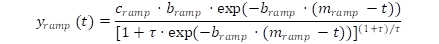(1)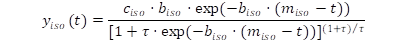(2)

where and represent the time elapsed from the beginning of the experiment to the instant where the maximum mass loss rate is observed, and are related to the rate of the process, and and represent the peak areas and are related to the mass loss involved in each degradation step. The τparameter in Eqs. 1 and 2 is related to the symmetry of the peak representing the process. Thus a value of τ =1 represents a perfectly symmetric peak, while values higher than 1 correspond to right-skewed peaks and tau values between 1 and 0 correspond to left-skewed peaks. In the context of thermal degradation, a left skewed peak usually appears in linear heating experiments where the degradation rate increases with time as a consequence of the increase of temperature until the unreacted fraction becomes too small. Similarly, a right-skewed peak usually appears in isothermal experiments where the maximum degradation rate is quickly reached and then continuously slows down as the unreacted fraction decreases. On the other hand, as explained in our previous works, Eqs. 1 and 2 can be written as a non-Arrhenius reaction order functions, where the reaction order is represented by 1+ τ (López-Beceiro et al. 2013, 2014). Generalised logistic functions were firstly used by the authors to represent a non-reversing process (enthalpic relaxation) in a linear heating ramp experiment (Artiaga et al. 2011). Equation 1 applies to the heating ramp context as denoted by the ramp subindex. Similarly, the iso subindex of Eqn. 2 refers to isothermal conditions. Although the generalised logistic function, also known as the Richard’s function, was broadly applied in different scientific fields with different aims, the use of that function and its time derivative in the context of our kinetic model was introduced in 2012 for the study of polymer crystallization from the molten state (López-Beceiro et al. 2013). The method for kinetic analysis consisted of fitting experimental DTG curves to mixtures of generalised logistics. Then, the parameter values resulting from isotherms at different temperatures and ramps at different heating rates were analysed together, through the relationships of the model proposed by the authors, to obtain two insightful parameters: a critical temperature, Tc, and an energy barrier, Eiso. The time derivative thermogravimetric data of each linear heating experiment was fitted by the sum of two components, P1 and P2, described by Eq 1. The Fityk software was used to optimize the fittings (Wojdyr 2010). The best-fit curve was assumed to be that which minimizes the sum of squared residuals. The parameter values were estimated by the non-linear least squares method, whose fundamentals were described by Gay (1984). The Nelder-Mead method for generation of the approximation sequence to the minimum point was used for the calculation of the parameter values that minimize that sum (Nelder and Mead 1965). The results of that fittings, displayed on Fig. 1, clearly show that one of the two components was negligible, being the process accurately represented by only one component. Thus, in the isothermal context, only one component represented by Eq. 2 was used to fit, using the same software and method than for the linear heating case, each of the time derivative thermogravimetric curves obtained in isothermal conditions. The range of DTG data used for the fittings in the linear heating cases included the complete mass loss process and a few data of the zero baseline before the beginning and after the end of the process. However, in the isothermal cases, the mass loss could start before reaching the isothermal condition. In that cases, the fittings covered a broad range of conversion: from near the beginning of the isotherm to a point where the DTG curve recovered a null mass loss rate or at a point, after more than 200 min from the beginning of the isothermal condition, where the mass loss rate was very low.

RESULTS AND DISCUSSION

Non-Isothermal Data

Figure 1 shows how all heating rate DTG curves were accurately fitted by the sum of two logistic derivative components, P1 and P2. The main degradation process was represented by one single component, P1, being the other, P2, practically negligible. This means that the degradation basically consists of a single process, which is accurately described by the logistic derivative component P1.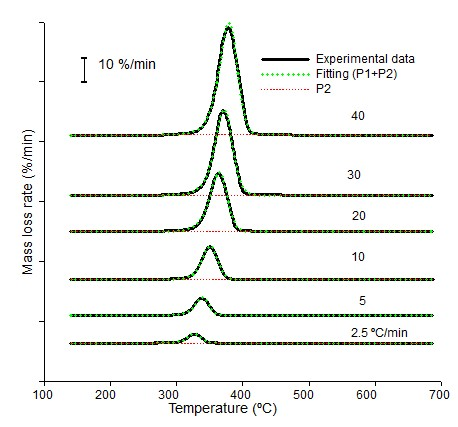Fig. 1. DTG curves obtained at the indicated heating rates and their corresponding fittings

Table 1 displays the optimal parameter values of the P1 component obtained at all heating rates. The determination index, R2, which is also displayed, was related to the quality of the fittings. As mentioned above, mramp represents the time elapsed from the beginning of the experiment to the instant where the maximum mass loss rate is observed. That values of mramp are affected by the start-up time, which may differ from one experiment to other and, thus, are not suitable to be compared among different experiments. However, the temperature at the mramp point is the peak temperature, Tp, and this is a very convenient parameter for comparison of the curves obtained at different heating rates.

Table 1. Optimal Parameter Values of the P1 Component Resulting from the Fittings of DTG Curves Obtained at Different Heating Rates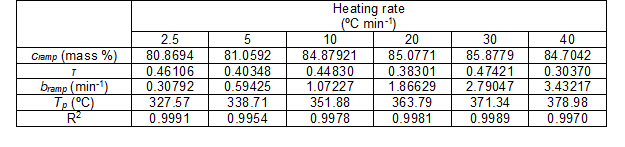Equation 1 can be expressed as a function of the degree of advancement of the process, α,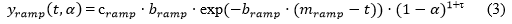where 1 + τ represents an order of reaction.

According to the model, the bramp parameter can be expressed as (López-Beceiro et al. 2013),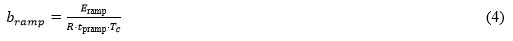where Tc is the critical temperature below which the process cannot practically occur and tpramprepresents the time elapsed from the instant when a critical value of temperature Tc is reached to the instant where the maximum rate of change mramp is observed. Eramp is an apparent energy barrier, and R is the gas constant. Tc and tpramp are related to each other through this expression,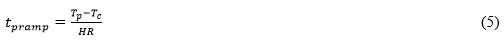where Tp is the peak temperature that can be taken from the single curve fittings of DTG curves and HR is the heating rate. Obviously, if a given process is accurately represented by the model, the plot of the bramp values, which resulted from the fittings of single curves versus 1/(R tprampTc), would be a straight line that passes through the origin and has a slope equal to Eramp. Starting with some pilot Eramp and Tc values, an iterative process made it possible to obtain the best fitting equation (Eq. 4). Figure 2 shows that the bramp values obtained from the fittings of single DTG curves obtained in the ramp nearly fell into a straight line, as predicted by the model. New brampvalues obtained from the straight line at the same x-axis position verified that this assumption did not appreciably decrease the quality of fittings of the single DTG curves, as demonstrated in Fig. 1. An apparent energy barrier of 56,503 J·mol -1 and a critical temperature of 226.6 °C were obtained.

A set of lines crossing at approximately T = 226.6 °C was obtained when plotting the exponential term of Eq. 3 versus temperature, as shown in Fig. 3. This is so because, according to Eq. 4, the product of bramp and the peak time is a constant and, on the other hand, the value of mramp-t at T=Tc is exactly the peak time, as defined for Eq. 3. For any other temperature, always in the linear heating context, mramp-t will be different than the peak time and this is why the exponential term varies with temperature. In some of our previous works, the temperature value at the crossing point was called critical temperature (López-Beceiro et al. 2013).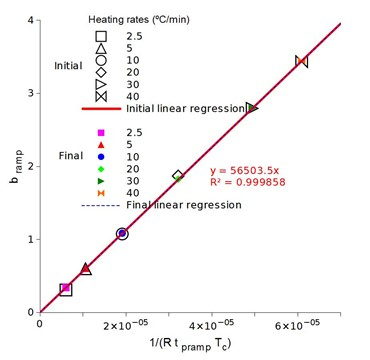Fig. 2. Plot of bramp parameters values obtained from the fittings of non-isothermal data versus 1/(R.tpramp.Tc)

One can say that cellulose can be safely preserved at any temperature below its critical temperature. But that does not mean that the mechanical properties are the same at any temperature, since they depend on the temperature. As a practical application, paper documents can be thermally treated to destroy mites or other biological agents, as long as one pays attention to Tc, the maximum temperature limit, and carries out the treatment in a nitrogen atmosphere for thermal stability of cellulose. However, in the case of wood, which is composed of several biopolymers different from cellulose, if some of that biopolymers have a lower Tc than cellulose, that Tc would be its limiting temperature.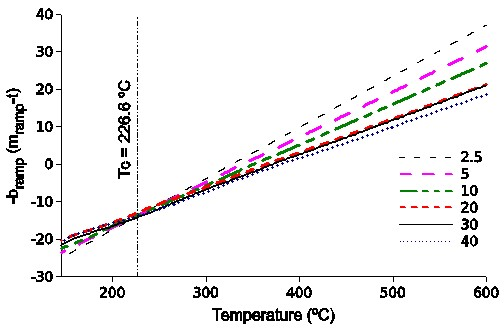Fig. 3. Plots of the exponential term of Eq. 3 versus temperature, corresponding to the fittings of experimental curves at the indicated heating rates

Isothermal Data

To isothermally determine the kinetic parameters of the P1 process, temperatures in the range from 280 to 300 °C were chosen. According to Fig. 1, P1 was the only significant process in that range of temperature. Thus, only one derivative logistic component was needed to fit each of the isothermal DTG curves. Table 2 shows the parameter values resulting from the optimal fittings. The peak time, tpiso, is calculated as the difference from the time at the beginning of the isotherm to miso (the time measured at the peak maximum).

Table 2. Parameter Values Obtained from the Fittings of Isothermal DTG Curves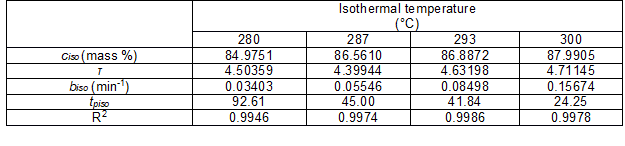Equation 2 can be written as functions of the progress variable of the process, α, taking the form of a reaction order model, where the reaction order, n, is represented by 1 + τ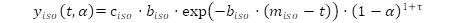(6)

This expression can be rewritten as (López-Beceiro et al. 2015),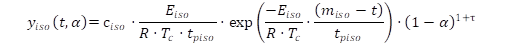(7)

where tpiso represents the peak time and Eiso has dimensions of enthalpy. Accordingly, a true Arrhenius dependence would only be observed at the beginning of a hypothetical isothermal case at T = Tc. We would like to point out that while Arrhenius-based models assume that the processes can take place at any temperature above 0 K, although at a very slow rate, the model used here assumes that there is a minimum temperature, Tc, below which the processes are prevented.

In a previous study (López-Beceiro et al. 2013), the following expressions were proposed for the peak time, tpiso, and for the biso parameter, which is related to the peak width: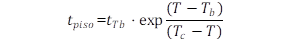(8)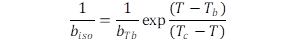(9)

In this context, Tc is the aforementioned critical temperature, and tTb and bTb are two constants representing the values of tpiso and biso, respectively, at Tb. On the other hand, Tb is a fitting parameter in Eqs. 8 and 9. It simply represents a reference temperature, higher than Tc, at which the reaction would be relatively fast.

In an ideal isothermal experiment, temperature would be instantaneously raised from below Tc to the programmed isothermal temperature. Then, the peak time, tpiso, the time from the instant where Tc to the instant where a maximum reaction rate is observed, is the time elapsed from the beginning of the isotherm to the peak. Nevertheless, in practice, it takes some time to raise the temperature from below Tc to the isothermal value. Consequently, measuring only the isothermal time implies underestimating the peak time. On the other hand, measuring the time from Tc would involve the error of counting a part of the pre-isothermal time, at which the reaction is expected to be slower than at the isothermal temperature, as a part of the isotherm. An additional difficulty consists of determining the maximum on a broad peak such as those obtained at relatively low temperatures.

Accepting that these slight errors may be involved, peak times were measured from the beginning of the isotherms. Making use of the Tc value calculated in the ramp, the biso values obtained from the fittings of the individual isothermal DTG curves, and the tpiso values measured from the beginning of the isotherms on the same DTG curves, a fitting was simultaneously performed on Eqs. 8 and 9 by minimising the weighted sum of squared residuals (WSSR). Table 3 shows the initial tpiso and biso values obtained from the fittings of experimental DTG curves along with those resulting from the fittings to Eqs. 8 and 9. The initial values were close to those of Eqs. 8 and 9.

Table 3. Initial tpiso and biso Values Obtained from the Fittings of Experimental DTG Curves Compared to Fittings to Eqs. 8 and 9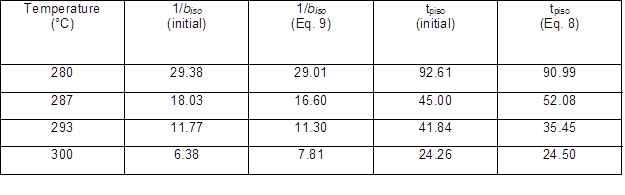The isothermal parameters are related through an expression, which is formally equivalent to Eq. 4: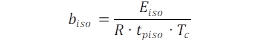(10)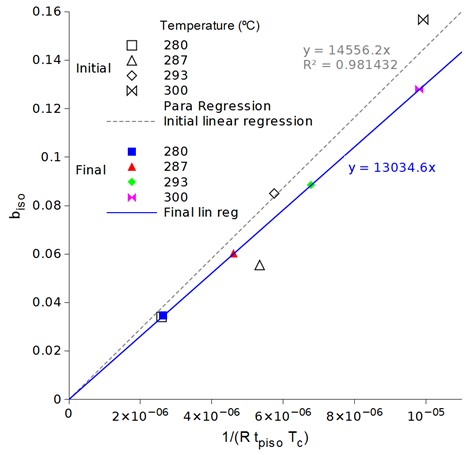Fig. 4. Plot of biso values versus 1/(R tpisoTc)

Eiso is the true energy barrier and is obtained as the slope of the linear fit when plotting the bisovalues versus 1/(R·tpiso·Tc). The value obtained was 13,035 J·mol-1. This value is much lower than the apparent energy barrier value obtained from linear heating data. It is not surprising that both values are totally different and cannot be compared to each other because the reaction rate depends on the temperature and, thus, isothermal and non-isothermal conditions result in different peak times. It is observed that when a linear heating is applied, the relation between the peak time and the b parameter (which is related to the maximum transformation rate) is different than in the case of isotherms. The biso values that resulted from the fittings of single isothermal curves approximately follow a linear trend line and are not very different from those obtained by simultaneous fitting to Eqs. 8 and 9, which fell exactly on a different straight line, as displayed in Fig. 4.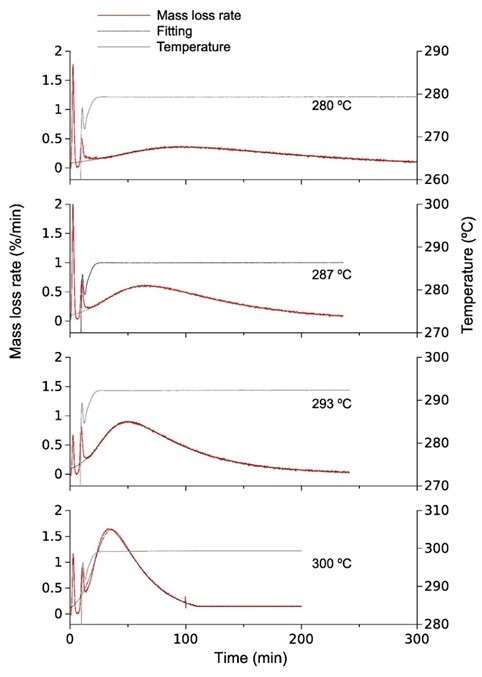Fig. 5. Plots of DTG curves and their corresponding fittings, obtained in isothermal experiments. The actual temperatures are also displayed.

The newly estimated biso values were taken for new fittings of the isothermal curves, allowing the other parameter values to vary. It can be clearly observed in Fig. 5 that the model very well fit the peak of all curves, which were primarily located in the isothermal region. The temperature profile is also displayed for clarity. Only the DTG data in the range where the isotherm was effectively kept were used for the calculations, discarding the small region previous to the isotherm.

Significance of Parameter Values

It was previously explained that τ is related to the asymmetry of the DTG peak, so that τ = 1 corresponds to a perfectly symmetric peak. A value higher than 1 corresponds to a peak with lower slope on the right side, that is, on the side of higher conversion. Also, 1 + τ represents a reaction order and the reaction rate is proportional to (1 – α)1+τ , where α is the conversion.

Nevertheless, when it comes to the thermal degradation of polymers, one cannot assume the physical meaning of a classical reaction order. That is because thermal degradation usually causes fragmentation, voids, and the emission of volatiles into the sample, which increasingly affect the mass transport phenomena as the reaction proceeds. Thus, the asymmetry of the curves and the reaction order values were probably affected by those fragmentation changes in the sample.

Tables 1 and 2 show how τ depends on the experimental conditions such as the heating rate and the isothermal temperature. In the case of heating rate, the values fell into the range between 0.3 and 0.5, while in the isotherms, the values ranged from 4.4 to 4.7. Fragmentation of the sample and the formation of voids would have more opportunity to arise during a slow process than in a fast one. Thus, the isotherms were obtained at low temperatures, compared with the peak temperatures observed in the ramps, and involved much longer degradation times and higher τvalues than the ramps.

Although the true energy barrier concept used here is similar to the energy barrier used by Arrhenius, their values are not equivalent to each other because they include different relationships: the reaction rate dependence on temperature assumed by both kinds of models is different. Thus, values of the Arrhenius energy barrier can only be compared with those obtained with models assuming the Arrhenius dependence on temperature. Energy barrier values obtained for other processes and materials, assuming the same model as in the present work, were recently reported by the authors (López-Beceiro et al. 2015; Zaragoza et al. 2015). In this context, the true energy barrier value obtained here, 13 kJ mol-1, was on the same order as those obtained for the main degradation step of some acrylic-based copolymers (approximately 11 kJ mol-1) and polyetherimide (22 kJ mol-1), as displayed in Table 4.

The Eramp/Eiso rate is related to the accelerating effect of the temperature on the reaction rate (López-Beceiro et al. 2014). Table 4 shows that the value obtained here, 4.3, was on the same order as that observed in the thermal degradation of other polymers.

Another insightful parameter for the linear heating experiments is the peak temperature, Tp. The distance of Tp from Tc followed a power trend with the heating rate, so that the peak temperature moved closer to the critical temperature as the heating rate decreased. This trend allows the determination of Tc by extrapolating T– T= 0, as shown in Fig. 6. On the other hand, in isothermal conditions, the peak time, tpiso, tends to infinity as the isothermal temperature, Tiso, approaches Tc. Figure 7 shows plots of Tiso – Tc versus the peak time using the same Tc value obtained in the ramp.

Table 4. Eramp/Eiso Values Obtained in Different Processes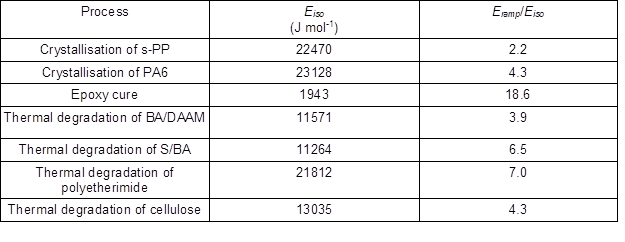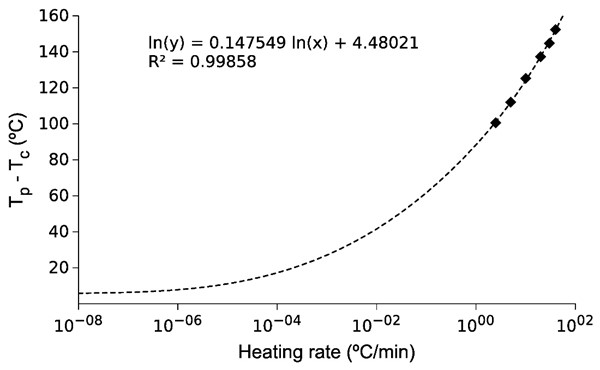Fig. 6. Plot of T– Tc versus the heating rate

Beyond the possibility of making predictions of the degradation rate and extent as a function of temperature and time, a few insights can be gained from looking at the main features of the model and the resulting parameter values: one is that the degradation process of cellulose is observed as a single peak, without shoulders, on the DTG curve and is accurately fitted by a single function. This suggests that the pyrolitic degradation of cellulose occurs as a single mass loss step process. In case there were several steps, these would be very strongly overlapping and, in any case, the overall process can be reproduced by a single function. These results are in line with those reported by Várhegyi et al. (1993), which assigns this rate determining reaction to the degradation of the cellulose to monomers and oligomers. But, when the transport of the volatiles from the sample is hindered, the mechanism becomes more complex (Várhegyi et al. 1993). On the other hand, the model used, which is of the reaction order type, provides better fittings than any other tested up to the moment.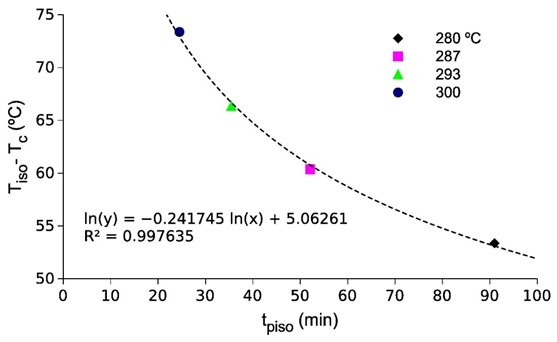Fig. 7. Plot of Tiso – Tc versus the Tpiso values obtained from the isothermal experiments

The apparent reaction order observed in isotherms is higher than the chemical reaction order probably because of voids formation and fragmentation effects. However, the reaction order values observed in ramp (with less opportunity of fragmentation and voids formation) are about 1.4 (since the reaction order is 1+τ). A fractional order reaction often indicates a chemical chain reaction or other complex reaction mechanism. For example, thermal degradation of acetaldehyde in nitrogen was reported to have a reaction order of 1.5, following the Rice-Herzfeld mechanism with homolytic scission producing radicals (Atkins and Paula 2006). This approach is in line with some of the early studies of cellulose pyrolysis (Tang and Bacon 1964; Chatterjee 1968). It is important to point out that the results of this work were obtained in a TG instrument using small samples in open crucibles and with continuous nitrogen flow. In these conditions, there is not likely to have the effect of confined self-generated volatiles which are normally present in closed reactors and which may drastically change the predominance of some chemical reactions with respect to others, adding more complexity to the decomposition mechanism.

CONCLUSIONS

1. Thermal degradation of cellulose in nitrogen atmosphere was accurately described by the model proposed by the authors.
2. Fittings of superior quality to any other model reported up to the moment were obtained in isothermal and linear heating experiments for the full extent of conversion.
3. The results from different experimental conditions accorded with each other, and were related through some kinetic parameters.
4. The critical temperature was 226.6 °C. The energy barrier value obtained from cellulose was in the middle of that obtained from other polymers. The apparent reaction order observed in isotherms is higher than the chemical reaction order, probably because of voids formation and fragmentation effects. However, the reaction order values observed in ramp, about 1.4, suggest a chemical chain reaction or other complex reaction mechanism.

ACKNOWLEDGMENTS

This work was partially funded by the Spanish Ministerio de Educación y Ciencia (MTM2011-22393 and MTM2014-52876-R).

REFERENCES CITED

Agrawal, R. K. (1988a). “Kinetics of reactions involved in pyrolysis of cellulose I. The three reaction model,” The Canadian Journal of Chemical Engineering 66(3), 403-412. DOI: 10.1002/cjce.5450660309

Agrawal, R. K. (1988b). “Kinetics of reactions involved in pyrolvsis of cellulose II. The modified Kilzer-bioid model,” The Canadian Journal of Chemical Engineering 66(3), 413-418. DOI: 10.1002/cjce.5450660310

Alwani, M. S., Khalil, H. P. S. A., Sulaiman, O., Islam, M. N., and Dungani, R. (2013). “An approach to using agricultural waste fibres in biocomposites application: Thermogravimetric analysis and activation energy study,” BioResources 9(1), 218-230. DOI: 10.15376/biores.9.1.218-230

Antal, M. J., and Varhegyi, G. (1995). “Cellulose pyrolysis kinetics: The current state of knowledge,” Industrial and Engineering Chemistry Research 34(3), 703-717. DOI: 10.1021/ie00042a001

Antal, M. J., Varhegyi, G., and Jakab, E. (1998). “Cellulose pyrolysis kinetics:  Revisited,” Industrial & Engineering Chemistry Research 37(4), 1267-1275. DOI: 10.1021/ie970144v

Artiaga, R., López-Beceiro, J., Tarrío-Saavedra, J., Gracia-Fernández, C., Naya, S., and Mier, J. L. (2011). “Estimating the reversing and non-reversing heat flow from standard DSC curves in the glass transition region,” Journal of Chemometrics 25(6), 287-294.

Atkins, P., and Paula, J. de. (2006). “The kinetics of complex reactions,” in: Physical Chemistry, 8th Ed., W. H. Freeman Ed., 830.

Bauer, W. D., Talmadge, K. W., Keegstra, K., and Albersheim, P. (1973). “The structure of plant cell walls II. The hemicellulose of the walls of suspension-cultured sycamore cells,” Plant Physiology 51(1), 174-187. DOI: 10.1104/pp.51.1.174

Bradbury, A. G. W., Sakai, Y., and Shafizadeh, F. (1979). “A kinetic model for pyrolysis of cellulose,” Journal of Applied Polymer Science 23(11), 3271-3280. DOI: 10.1002/app.1979.070231112

Burnham, A. K., Zhou, X., and Broadbelt, L. J. (2015). “Critical review of the global chemical kinetics of cellulose thermal decomposition,” Energy and Fuels 29(5), 2906-2918. DOI: 10.1021/acs.energyfuels.5b00350

Capart, R., Khezami, L., and Burnham, A. K. (2004). “Assessment of various kinetic models for the pyrolysis of a microgranular cellulose,” Thermochimica Acta 417(1), 79-89. DOI: 10.1016/j.tca.2004.01.029

Chatterjee, P. K. (1968). “Chain reaction mechanism of cellulose pyrolysis,” Journal of Applied Polymer Science 12(8), 1859-1864. DOI: 10.1002/app.1968.070120807

Chen, Z., Hu, M., Zhu, X., Guo, D., Liu, S., Hu, Z., Xiao, B., Wang, J., and Laghari, M. (2015). “Characteristics and kinetic study on pyrolysis of five lignocellulosic biomass via thermogravimetric analysis,” Bioresource Technology 192, 441-450. DOI: 10.1016/j.biortech.2015.05.062

Ding, Y., Wang, C., Chaos, M., Chen, R., and Lu, S. (2016). “Estimation of beech pyrolysis kinetic parameters by shuffled complex evolution,” Bioresource Technology 200, 658-665. DOI: 10.1016/j.biortech.2015.10.082

Dumitriu, S. (ed.) (2004). Polysaccharides: Structural Diversity and Functional Versatility, 2ndEd., CRC Press, New York, NY.

Gay, D. M. (1984). “A trust-region approach to linearly constrained optimization,” in: Numerical Analysis, D. F. Griffiths (ed.), Springer Berlin Heidelberg, 72–105.

Henrique, M. A., Neto, W. P. F., Silvério, H. A., Martins, D. F., Gurgel, L. V. A., Barud, H. D. S., Morais, L. C. D., and Pasquini, D. (2015). “Kinetic study of the thermal decomposition of cellulose nanocrystals with different polymorphs, cellulose I and II, extracted from different sources and using different types of acids,” Industrial Crops and Products 76, 128-140. DOI: 10.1016/j.indcrop.2015.06.048

Janković, B. (2014). “The pyrolysis process of wood biomass samples under isothermal experimental conditions—energy density considerations: Application of the distributed apparent activation energy model with a mixture of distribution functions,” Cellulose 21(4), 2285-2314. DOI: 10.1007/s10570-014-0263-x

Khawam, A., and Flanagan, D. R. (2005a). “Role of isoconversional methods in varying activation energies of solid-state kinetics: I. Isothermal kinetic studies,” Thermochimica Acta429(1), 93-102. DOI: 10.1016/j.tca.2004.11.030

Khawam, A., and Flanagan, D. R. (2005b). “Role of isoconversional methods in varying activation energies of solid-state kinetics: II. Nonisothermal kinetic studies,” Thermochimica Acta436(1-2), 101-112. DOI: 10.1016/j.tca.2005.05.015

Liu, H., Liu, D., Yao, F., and Wu, Q. (2010). “Fabrication and properties of transparent polymethylmethacrylate/cellulose nanocrystals composites,” Bioresource Technology 101(14), 5685-5692. DOI: 10.1016/j.biortech.2010.02.045

López-Beceiro, J., Gracia-Fernández, C., and Artiaga, R. (2013). “A kinetic model that fits nicely isothermal and non-isothermal bulk crystallizations of polymers from the melt,” European Polymer Journal 49(8), 2233-2246. DOI: 10.1016/j.eurpolymj.2013.04.026

López-Beceiro, J., Fontenot, S. A., Gracia-Fernández, C., Artiaga, R., and Chartoff, R. (2014). “A logistic kinetic model for isothermal and nonisothermal cure reactions of thermosetting polymers,” Journal of Applied Polymer Science 131(17), 40670. DOI: 10.1002/app.40670

López-Beceiro, J., Álvarez-García, A., Martins, S., Álvarez-García, B., Zaragoza-Fernández, S., Menéndez-Valdés, J., and Artiaga, R. (2015). “Thermal degradation kinetics of two acrylic-based copolymers,” Journal of Thermal Analysis and Calorimetry 119(3), 1981-1993. DOI: 10.1007/s10973-014-4386-y

Mamleev, V., Bourbigot, S., and Yvon, J. (2007a). “Kinetic analysis of the thermal decomposition of cellulose: The change of the rate limitation,” Journal of Analytical and Applied Pyrolysis80(1), 141-150. DOI: 10.1016/j.jaap.2007.01.012

Mamleev, V., Bourbigot, S., and Yvon, J. (2007b). “Kinetic analysis of the thermal decomposition of cellulose: The main step of mass loss,” Journal of Analytical and Applied Pyrolysis 80(1), 151-165. DOI: 10.1016/j.jaap.2007.01.013

Mamleev, V., Bourbigot, S., Le Bras, M., and Yvon, J. (2009). “The facts and hypotheses relating to the phenomenological model of cellulose pyrolysis,” Journal of Analytical and Applied Pyrolysis 84(1), 1-17. DOI: 10.1016/j.jaap.2008.10.014

Manyà, J. J., Velo, E., and Puigjaner, L. (2003). “Kinetics of biomass pyrolysis: A reformulated three-parallel-reactions model,” Industrial and Engineering Chemistry Research 42(3), 434-441. DOI: 10.1021/ie020218p

McKendry, P. (2002). “Energy production from biomass (part 1): Overview of biomass,” Bioresource Technology 83(1), 37-46. DOI: 10.1016/S0960-8524(01)00118-3

Nelder, J. A., and Mead, R. (1965). “A simplex method for function minimization,” The Computer Journal 7(4), 308-313. DOI: 10.1093/comjnl/7.4.308

Orfão, J. J. M., Antunes, F. J. A., and Figueiredo, J. L. (1999). “Pyrolysis kinetics of lignocellulosic materials—Three independent reactions model,” Fuel 78(3), 349-358. DOI: 10.1016/S0016-2361(98)00156-2

Saddawi, A., Jones, J. M., Williams, A., and Wójtowicz, M. A. (2010). “Kinetics of the thermal decomposition of biomass,” Energy and Fuels 24(2), 1274-1282. DOI: 10.1021/ef900933k

Sánchez-Jiménez, P. E., Pérez-Maqueda, L. A., Perejón, A., and Criado, J. M. (2013). “Generalized master plots as a straightforward approach for determining the kinetic model: The case of cellulose pyrolysis,” Thermochimica Acta 552, 54-59. DOI: 10.1016/j.tca.2012.11.003

Sánchez-Jiménez, P. E., Pérez-Maqueda, L. A., Perejón, A., Pascual-Cosp, J., Benítez-Guerrero, M., and Criado, J. M. (2011). “An improved model for the kinetic description of the thermal degradation of cellulose,” Cellulose 18(6), 1487-1498. DOI: 10.1007/s10570-011-9602-3

Şerbănescu, C. (2014). “Kinetic analysis of cellulose pyrolysis: A short review,” Chemical Papers 68(7), 847-860. DOI: 10.2478/s11696-013-0529-z

Sfakiotakis, S., and Vamvuka, D. (2015). “Development of a modified independent parallel reactions kinetic model and comparison with the distributed activation energy model for the pyrolysis of a wide variety of biomass fuels,” Bioresource Technology 197, 434-442. DOI: 10.1016/j.biortech.2015.08.130

Shen, D. K., and Gu, S. (2009). “The mechanism for thermal decomposition of cellulose and its main products,” Bioresource Technology 100(24), 6496-6504. DOI: 10.1016/j.biortech.2009.06.095

Tang, M. M., and Bacon, R. (1964). “Carbonization of cellulose fibers-I. Low temperature pyrolysis,” Carbon 2(3), 211-214, IN1, 215-220.

Varhegyi, G., Antal Jr., M. J., Szekely, T., and Szabo, P. (1989). “Kinetics of the thermal decomposition of cellulose, hemicellulose, and sugarcane bagasse,” Energy and Fuels 3(3), 329-335. DOI: 10.1021/ef00015a012

Várhegyi, G., Szabó, P., Mok, W. S.-L., and Antal, M. J. (1993). “Kinetics of the thermal decomposition of cellulose in sealed vessels at elevated pressures. Effects of the presence of water on the reaction mechanism,” Journal of Analytical and Applied Pyrolysis, 26(3), 159-174. DOI: 10.1016/0165-2370(93)80064-7

Varhegyi, G., Jakab, E., and Antal Jr., M. J. (1994). “Is the Broido-Shafizadeh model for cellulose pyrolysis true?” Energy and Fuels 8(6), 1345-1352. DOI: 10.1021/ef00048a025

Várhegyi, G., Antal Jr., M. J., Jakab, E., and Szabó, P. (1997). “Kinetic modeling of biomass pyrolysis,” Journal of Analytical and Applied Pyrolysis 42(1), 73-87. DOI: 10.1016/S0165-2370(96)00971-0

Varhegyi, G., Grønli, M. G., and Blasi, C. D. (2004). “Effects of sample origin, extraction, and hot-water washing on the devolatilization kinetics of chestnut wood,” Industrial and Engineering Chemistry Research 43(10), 2356-2367. DOI: 10.1021/ie034168f

Wojdyr, M. (2010). “Fityk: A general-purpose peak fitting program,” Journal of Applied Crystallography 43(5), 1126-1128. DOI: 10.1107/S0021889810030499

Yahiaoui, M., Hadoun, H., Toumert, I., and Hassani, A. (2015). “Determination of kinetic parameters of Phlomis bovei de Noé using thermogravimetric analysis,” Bioresource Technology196, 441-447. DOI: 10.1016/j.biortech.2015.07.082

Yang, H., Yan, R., Chin, T., Liang, D. T., Chen, H., and Zheng, C. (2004). “Thermogravimetric analysis − Fourier transform infrared analysis of palm oil waste pyrolysis,” Energy & Fuels 18(6), 1814-1821. DOI: 10.1021/ef030193m

Zakikhani, P., Zahari, R., Sultan, M. T. H., and Majid, D. L. (2016). “Thermal degradation of four bamboo species,” BioResources 11(1), 414-425. DOI: 10.15376/biores.11.1.414-425

Zaragoza, S., Álvarez, A., Álvarez, B., López-Beceiro, J., Naya, S., Forcén, P., and Artiaga, R. (2015). “Thermogravimetric study of thermal degradation of polyetherimide,” Journal of Applied Polymer Science 132(31). DOI: 10.1002/app.42329

Article submitted: January 16, 2016; Peer review completed: February 27, 2016; Revised version received and accepted: May 3, 2016; Published: May 16, 2016.

DOI: 10.15376/biores.11.3.5870-5888